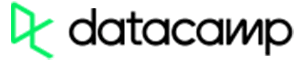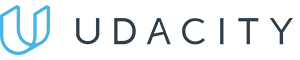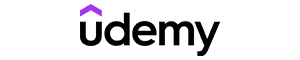Code has been added to clipboard!

# Everything You Need to Know About the Python Range

Published Oct 1, 2019
Updated Oct 3, 2019

TL;DR – Python `range()` is used for generating a sequence of numbers. It takes a starting point and ending point, and generates all the integers in between them.

## Basic syntax for Python range()

The `range()` function follows this syntax:

Example
``range([start,] stop [, step])``

The `start`, `stop`, and `step` parameters are all integers.

`start` and `stop` tell Python the numbers to start and stop generating integers at. The `step` parameter indicates whether numbers should be generated in sequence, or whether some specific integers should be skipped.

Note: only the stop parameter is required for the range() function to work. If you don’t provide a start value, Python will simply use zero.

To add one more number to the sequence, you need to raise the `stop` value by the amount of the `step` parameter. In the example below, the `step` value is not defined explicitly, so it's `1` by default. The current output is `0, 1, 2, 3`.

Note: the stop value is not included in the generated range.

If you want the function to generate a `4` as well, increase the `stop` value from `4` to `5`:

Example
``````for i in range(4):
print(i, end=', ')``````

Example
``0, 1, 2, 3,``

Python `range()` only supports integers by default. If you want to create a sequence of float values, you’ll need to create a new version of the `range()`function:

Example
``````def frange(start, stop, step):
i = start
while i < stop:
yield i
i += step

for i in frange(0.5, 1.0, 0.1):
print(i)``````

Example
``````0.5
0.6
0.7
0.7999999999999999
0.8999999999999999
0.9999999999999999``````

With this new function, you can create ranges of floating values.

Note: you can use other functions, such as len(), to specify the start, stop, or step parameters based on the length or other characteristics of another Python object.

## Understanding the step parameter

The `step` parameter is used to specify the numeric distance between each integer generated in the range sequence. By default, `range` in Python uses a `step` value of `1`.

If you want to generate a range that increases in increments greater than `1`, you’ll need to specify the `step` parameter. For example, to generate a range from `0` to `10` by increments of `2`, you would use:

Example
``range(0, 10, 2)``

The `step` parameter can also be used to generate a sequence that counts down rather than up. In that case, you need to reverse the `start` and `stop` values as well as specify a negative number for the `step` parameter:

Example
``range(10, 0, -2)``

Depending on your `start`, `stop`, and `step` values, it is also possible to generate a sequence that goes from negative to positive integers or from positive to negative integers:

Example
``range(-5, 5, 1)``
Example
``range(4, -2, -2)``Pros
• Easy to use with a learn-by-doing approach
• Offers quality content
• Gamified in-browser coding experience
• The price matches the quality
• Suitable for learners ranging from beginner to advanced
Main Features
• Free certificates of completion
• Focused on data science skills
• Flexible learning timetablePros
• Simplistic design (no unnecessary information)
• High-quality courses (even the free ones)
• Variety of features
Main Features
• Nanodegree programs
• Suitable for enterprises
• Paid Certificates of completionPros
• Easy to navigate
• No technical issues
• Seems to care about its users
Main Features
• Huge variety of courses
• 30-day refund policy
• Free certificates of completion

## Making a list of integers

Lists are extremely useful containers for data of different types in Python. How do you make a list of integers using `range()` in Python 3? Simply combine the `list()` command with the `range()` command:

Example
``print(list(range(10)))``

Example
``[0, 1, 2, 3, 4, 5, 6, 7, 8, 9]``

## Using Python range() in a for loop

One of the most common uses of the `range()` function is for iterating over a series of values in a `for` loop. This is particularly useful if you want to access each of the values in a list or array, or, for example, only every other value.

To give an example of how `range()` works with `for` loops, let’s make a list of integers and then double every value in the list:

Example
``````samples = [1, 3, 5, 7, 9]
for i in range(len(samples)):
print("Element index[", i, "]", "Previous value ", samples[i], "Now ", samples[i] * 2)``````

Example
``````Element index[ 0 ] Previous value  1 Now  2
Element index[ 1 ] Previous value  3 Now  6
Element index[ 2 ] Previous value  5 Now  10
Element index[ 3 ] Previous value  7 Now  14
Element index[ 4 ] Previous value  9 Now  18``````

In this example, the `range()` function is generating a sequence from `0` to `4`. The `for` loop is then using each value generated by `range()`, one at a time, to access a value from the `sampleList`.

This brings up an important point about the way `range()` differs in Python 2 and Python 3. In Python 3, the `range()` function generates each number in the sequence one at a time. That means it iterates through the sequence it is generating, rather than generating a list of integers at once. In Python 2, the `range()` function generated a list of integers automatically.

Days
Hours
Minutes
Seconds